Скачать презентацию Homework Reading Tokheim Chapter 9 1

2c88e5c16c9d97b28856004feaa3bbdf.ppt

• Количество слайдов: 20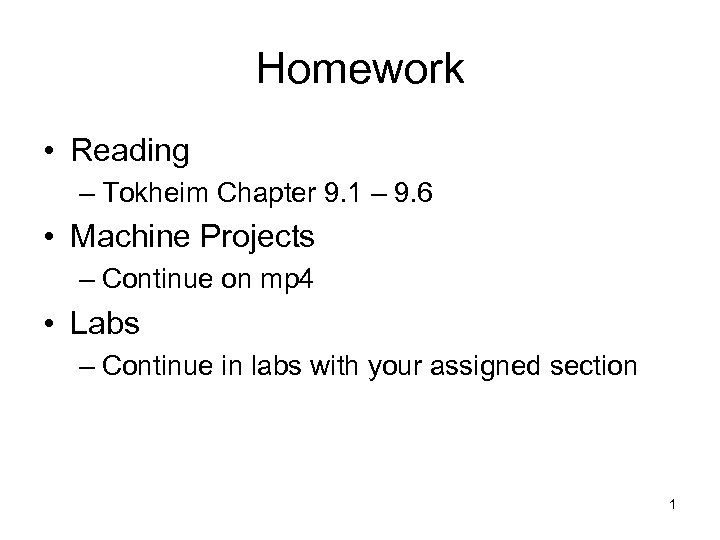Homework • Reading – Tokheim Chapter 9. 1 – 9. 6 • Machine Projects – Continue on mp 4 • Labs – Continue in labs with your assigned section 1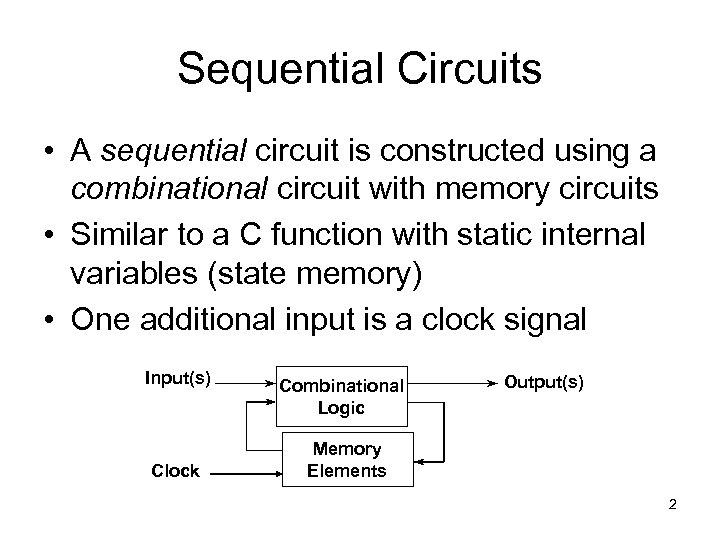Sequential Circuits • A sequential circuit is constructed using a combinational circuit with memory circuits • Similar to a C function with static internal variables (state memory) • One additional input is a clock signal Input(s) Clock Combinational Logic Output(s) Memory Elements 2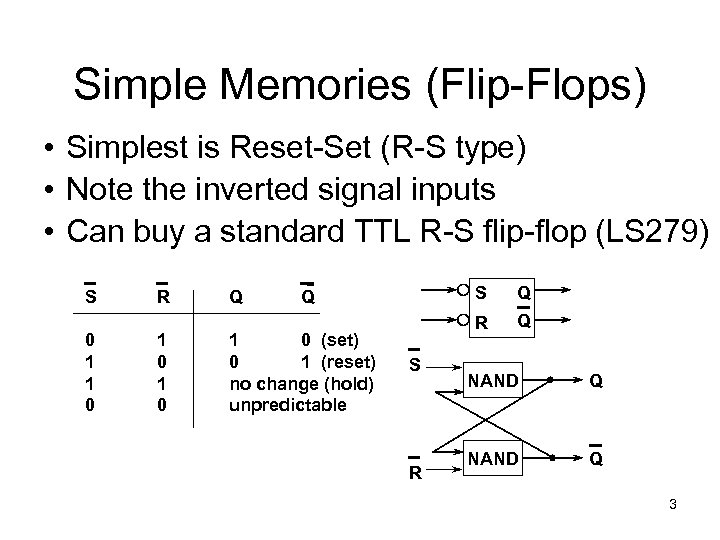Simple Memories (Flip-Flops) • Simplest is Reset-Set (R-S type) • Note the inverted signal inputs • Can buy a standard TTL R-S flip-flop (LS 279) S 0 1 1 0 R 1 0 Q S 1 0 (set) 0 1 (reset) no change (hold) unpredictable S R Q Q NAND Q 3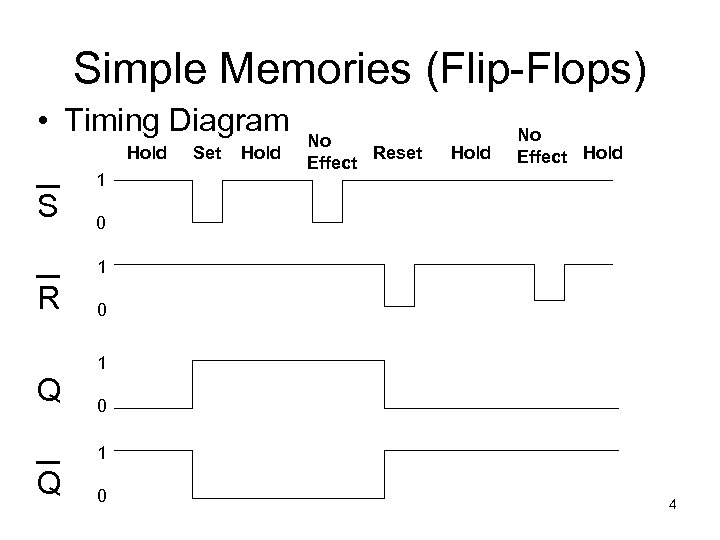Simple Memories (Flip-Flops) • Timing Diagram Hold S 1 Set Hold No Reset Effect Hold No Effect Hold 0 1 R Q 0 1 Q 0 4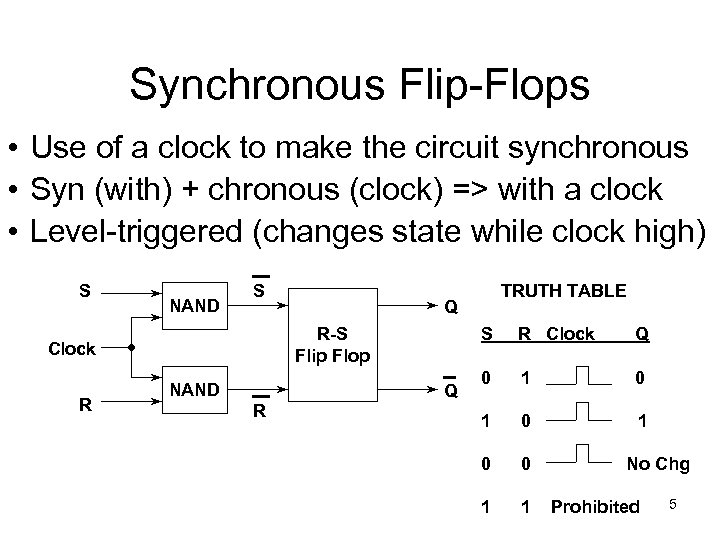Synchronous Flip-Flops • Use of a clock to make the circuit synchronous • Syn (with) + chronous (clock) => with a clock • Level-triggered (changes state while clock high) S NAND S R Q S R-S Flip Flop Clock NAND Q R TRUTH TABLE R Clock Q 0 1 0 1 0 0 1 1 No Chg Prohibited 5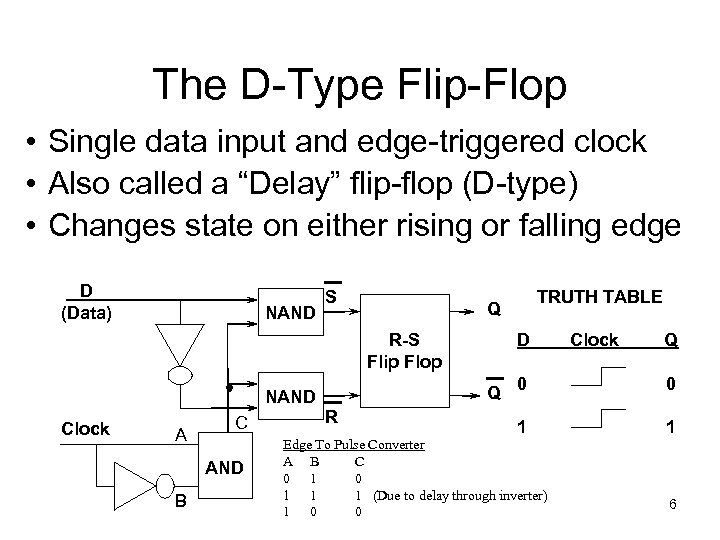The D-Type Flip-Flop • Single data input and edge-triggered clock • Also called a “Delay” flip-flop (D-type) • Changes state on either rising or falling edge D (Data) NAND S D R-S Flip Flop Q NAND Clock A C AND B R TRUTH TABLE Q Clock Q 0 0 1 1 Edge To Pulse Converter A B C 0 1 1 1 (Due to delay through inverter) 1 0 0 6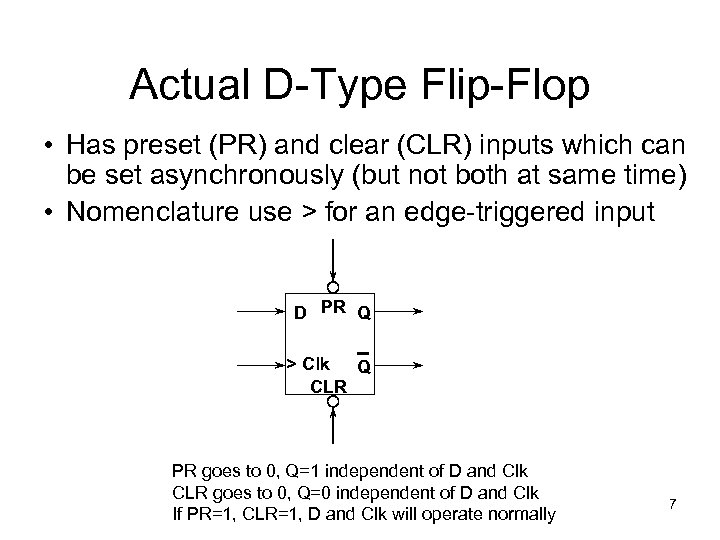Actual D-Type Flip-Flop • Has preset (PR) and clear (CLR) inputs which can be set asynchronously (but not both at same time) • Nomenclature use > for an edge-triggered input D PR Q > Clk Q CLR PR goes to 0, Q=1 independent of D and Clk CLR goes to 0, Q=0 independent of D and Clk If PR=1, CLR=1, D and Clk will operate normally 7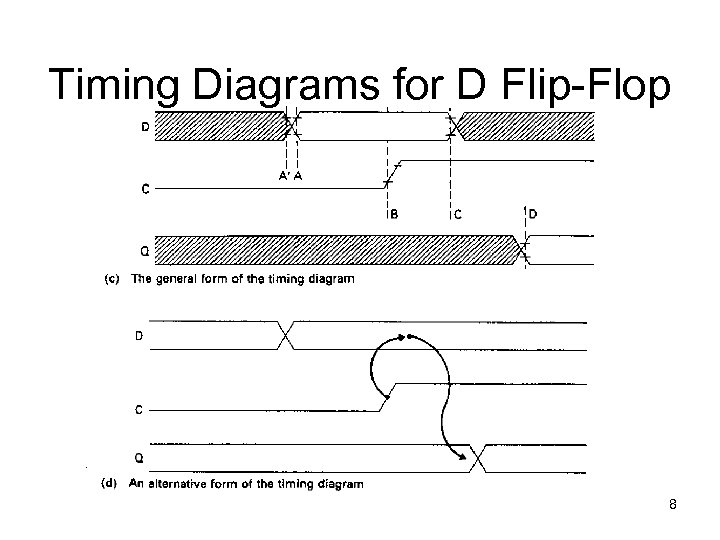Timing Diagrams for D Flip-Flop 8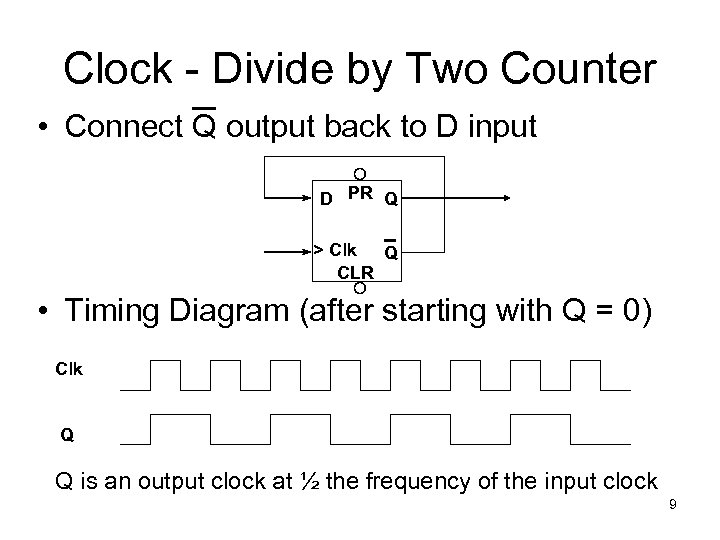Clock - Divide by Two Counter • Connect Q output back to D input D PR Q > Clk Q CLR • Timing Diagram (after starting with Q = 0) Clk Q Q is an output clock at ½ the frequency of the input clock 9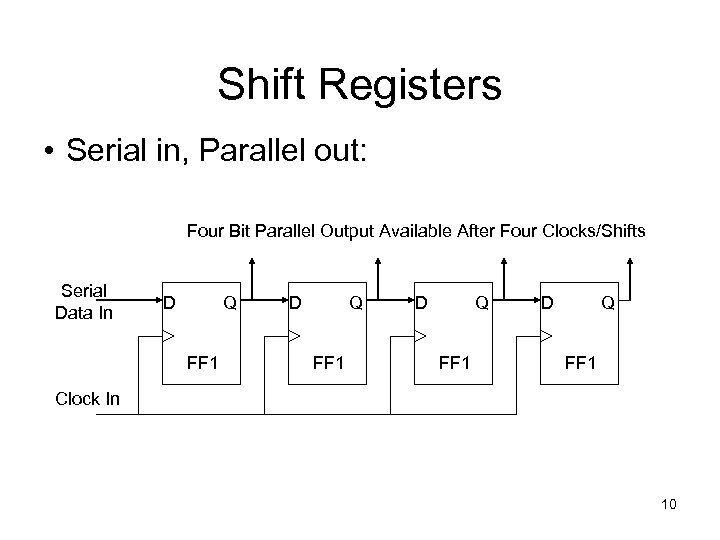Shift Registers • Serial in, Parallel out: Four Bit Parallel Output Available After Four Clocks/Shifts Serial Data In D Q FF 1 Clock In 10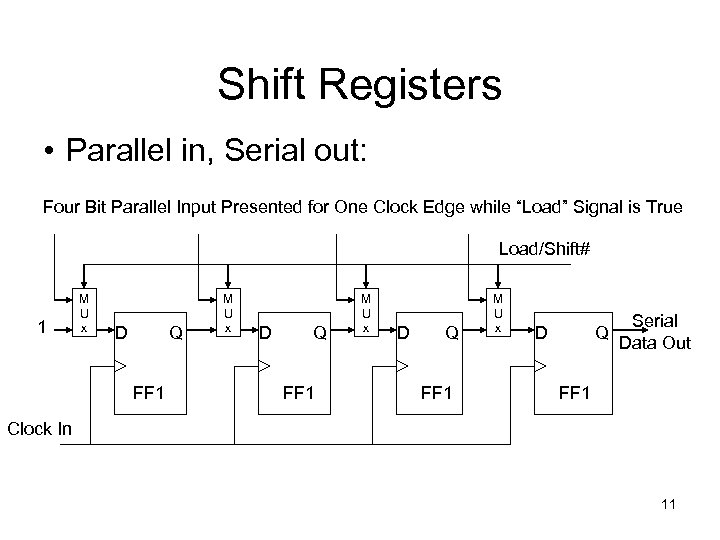Shift Registers • Parallel in, Serial out: Four Bit Parallel Input Presented for One Clock Edge while “Load” Signal is True Load/Shift# 1 M U x D Q FF 1 M U x D Q Serial Data Out FF 1 Clock In 11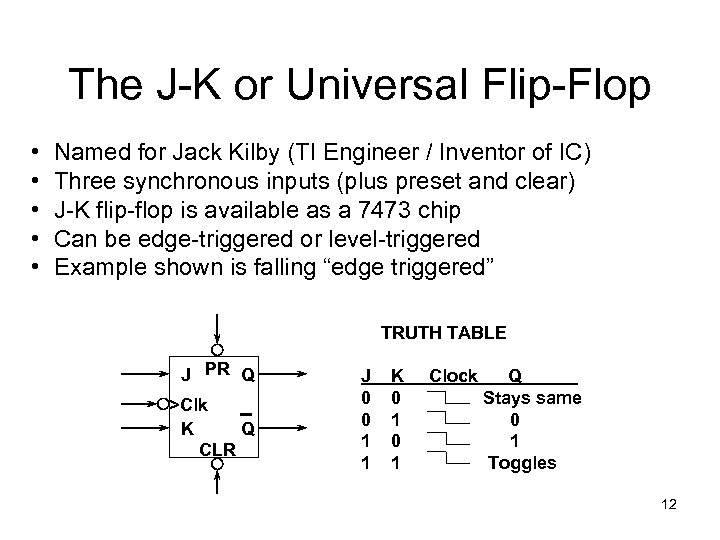The J-K or Universal Flip-Flop • • • Named for Jack Kilby (TI Engineer / Inventor of IC) Three synchronous inputs (plus preset and clear) J-K flip-flop is available as a 7473 chip Can be edge-triggered or level-triggered Example shown is falling “edge triggered” TRUTH TABLE J PR Q >Clk K Q CLR J 0 0 1 1 K 0 1 Clock Q Stays same 0 1 Toggles 12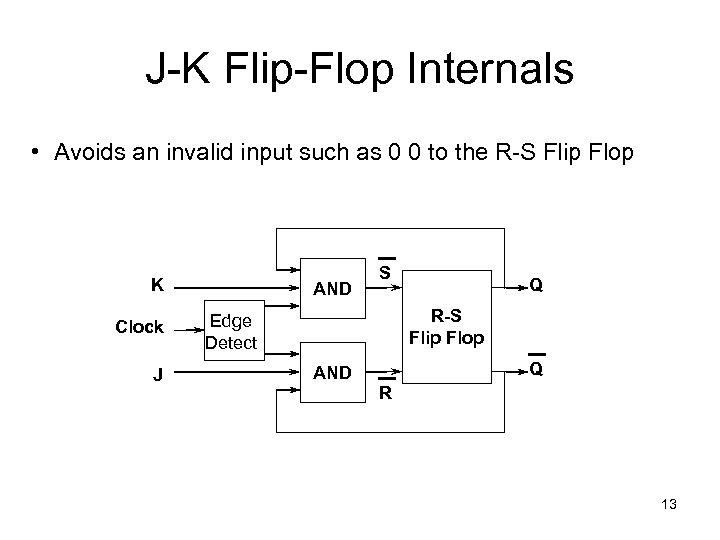J-K Flip-Flop Internals • Avoids an invalid input such as 0 0 to the R-S Flip Flop K Clock J AND S Q R-S Flip Flop Edge Detect Q AND R 13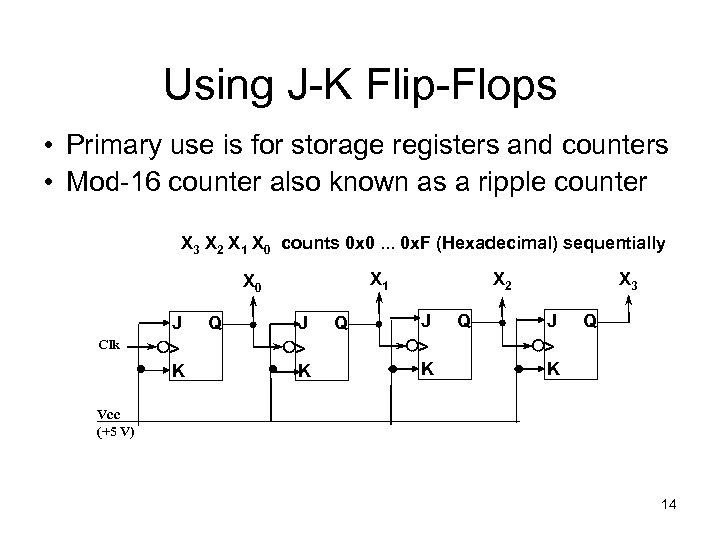Using J-K Flip-Flops • Primary use is for storage registers and counters • Mod-16 counter also known as a ripple counter X 3 X 2 X 1 X 0 counts 0 x 0. . . 0 x. F (Hexadecimal) sequentially X 1 X 0 Clk J > K Q X 2 J > K Q X 3 J > K Q Vcc (+5 V) 14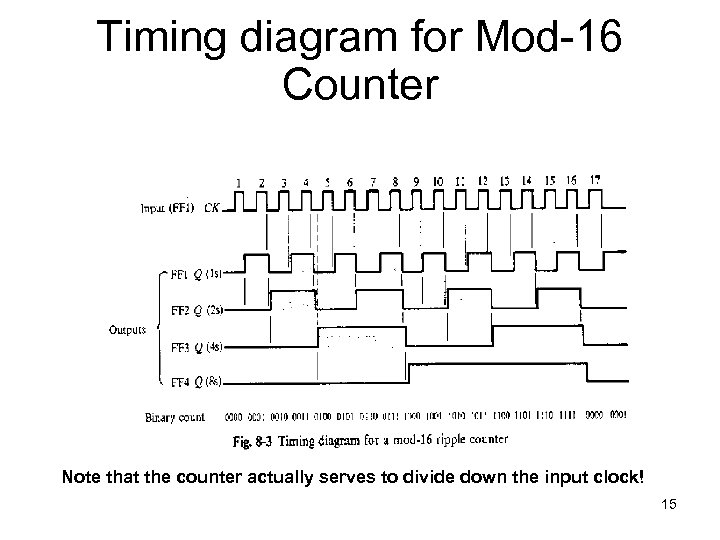Timing diagram for Mod-16 Counter Note that the counter actually serves to divide down the input clock! 15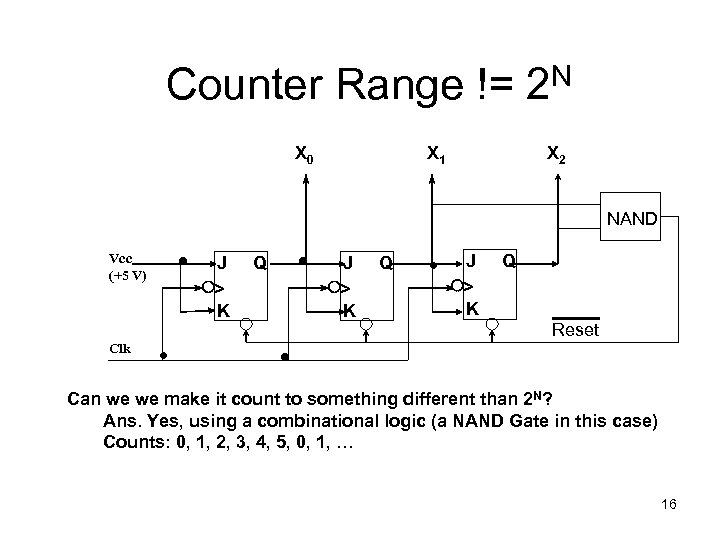Counter Range != 2 N X 0 X 1 X 2 NAND Vcc (+5 V) J > K Q Reset Clk Can we we make it count to something different than 2 N? Ans. Yes, using a combinational logic (a NAND Gate in this case) Counts: 0, 1, 2, 3, 4, 5, 0, 1, … 16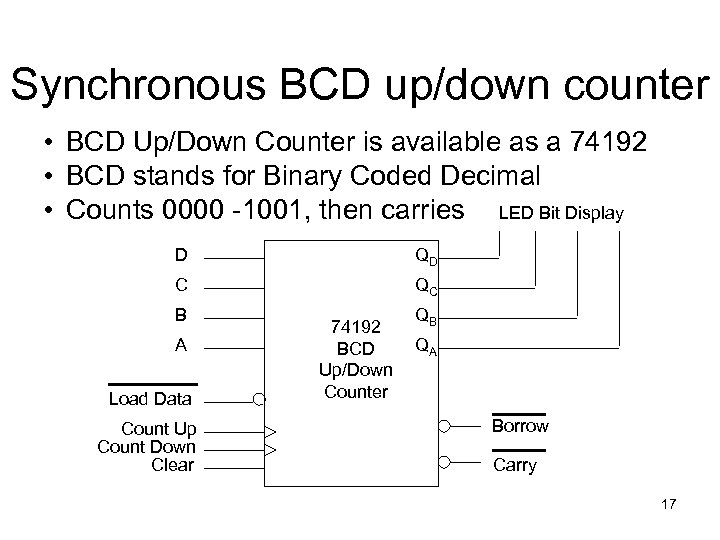Synchronous BCD up/down counter • BCD Up/Down Counter is available as a 74192 • BCD stands for Binary Coded Decimal • Counts 0000 -1001, then carries LED Bit Display D QD C QC B A Load Data Count Up Count Down Clear 74192 BCD Up/Down Counter QB QA Borrow Carry 17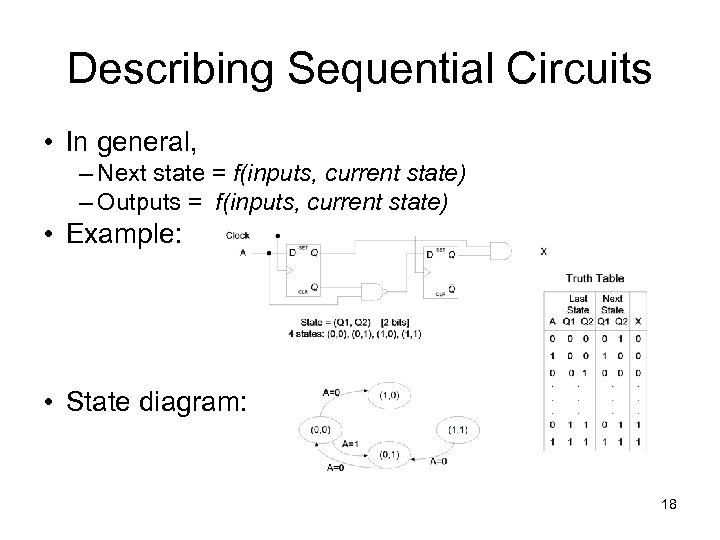Describing Sequential Circuits • In general, – Next state = f(inputs, current state) – Outputs = f(inputs, current state) • Example: • State diagram: 18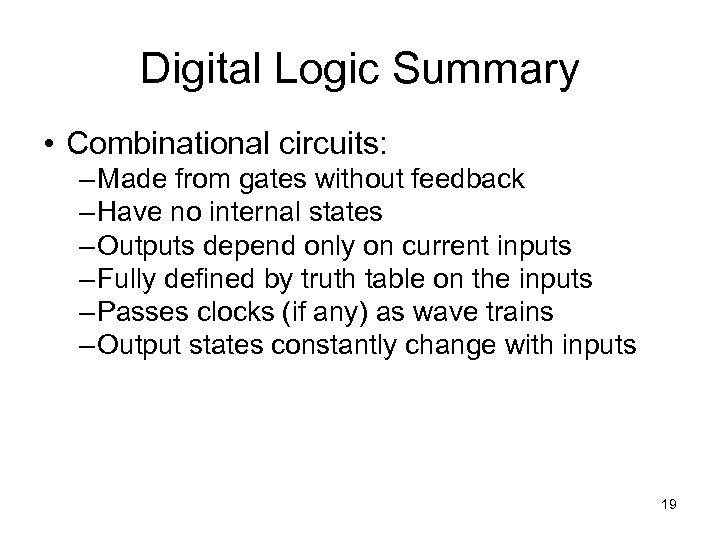Digital Logic Summary • Combinational circuits: – Made from gates without feedback – Have no internal states – Outputs depend only on current inputs – Fully defined by truth table on the inputs – Passes clocks (if any) as wave trains – Output states constantly change with inputs 19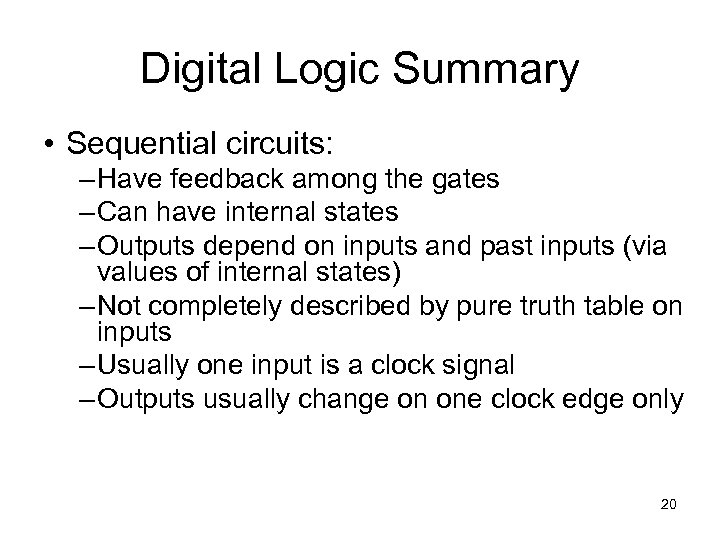Digital Logic Summary • Sequential circuits: – Have feedback among the gates – Can have internal states – Outputs depend on inputs and past inputs (via values of internal states) – Not completely described by pure truth table on inputs – Usually one input is a clock signal – Outputs usually change on one clock edge only 20